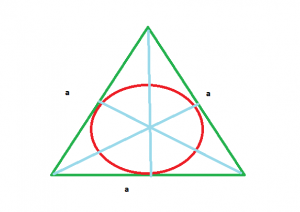# Area of circle which is inscribed in equilateral triangle

Given here is an equilateral triangle with side length a, the task is to find the area of the circle inscribed in that equilateral triangle.

Examples:

Input : a = 4
Output : 4.1887902047863905

Input : a = 10
Output : 26.1799387799


## Recommended: Please try your approach on {IDE} first, before moving on to the solution.Approach:

Area of equilateral triangle =Semi perimeter of equilateral triangle = (a + a + a) / 2

Radius of inscribed circle r = Area of equilateral triangle / Semi perimeter of equilateral triangle
==Area of circle = PI*(r*r) =Below is the implementation of above approach:

## C++

 // C++ program to find the area   // of circle which is inscribed  // in equilateral triangle   # include  # define PI 3.14  using namespace std;     // Function return the area of circle   // inscribed in equilateral triangle   float circle_inscribed(int a)  {      return PI * (a * a) / 12;  }     // Driver code   int main()  {      int a = 4;         cout << circle_inscribed(a);      return 0;  }     // This code is contributed   // by Mahadev99

## Java

 // Java program to find the area   // of circle which is inscribed  // in equilateral triangle   import java.io.*;     class GFG   {     static double PI = 3.14;     // Function return the area of circle   // inscribed in equilateral triangle   static double circle_inscribed(int a)  {      return PI * (a * a) / 12;  }     // Driver code   public static void main (String[] args)   {      int a = 4;         System.out.println(circle_inscribed(a));  }  }     // This code is contributed by anuj_67

## Python3

 # Python3 program to find the area of circle  # which is inscribed in equilateral triangle     # import math library for pi value  from math import pi     # Function return the area of circle  # inscribed in equilateral triangle  def circle_inscribed(a):      return pi*(a * a) / 12    # Driver code  a = 4 print(circle_inscribed(a))

## C#

 // C# program to find the area   // of circle which is inscribed  // in equilateral triangle   using System;     class GFG   {  static double PI = 3.14;     // Function return the area of circle   // inscribed in equilateral triangle   static double circle_inscribed(int a)  {      return PI * (a * a) / 12;  }     // Driver code   public static void Main ()  {      int a = 4;         Console.WriteLine( circle_inscribed(a));  }  }     // This code is contributed   // by inder_verma

## PHP

 

Output:

4.1887902047863905`

Don’t stop now and take your learning to the next level. Learn all the important concepts of Data Structures and Algorithms with the help of the most trusted course: DSA Self Paced. Become industry ready at a student-friendly price.

My Personal Notes arrow_drop_upCheck out this Author's contributed articles.

If you like GeeksforGeeks and would like to contribute, you can also write an article using contribute.geeksforgeeks.org or mail your article to contribute@geeksforgeeks.org. See your article appearing on the GeeksforGeeks main page and help other Geeks.

Please Improve this article if you find anything incorrect by clicking on the "Improve Article" button below.